The Big Bang

Start this free course now. Just create an account and sign in. Enrol and complete the course for a free statement of participation or digital badge if available.

Free course

# 5 The microwave background radiation

## 5.1 A second major discovery

In the introduction to this course, we said that there were three pillars of evidence for the big bang. We now turn to the second. It rests on a discovery that ranks in importance with that of Hubble's law. It came about when observations in a new region of the electromagnetic spectrum – the microwave region – became possible. This was due to the invention of new detectors, working at frequencies as high as 30 000 MHz. In 1965, two Bell Telephone scientists, A. Penzias and R. Wilson, were investigating the radio noise found at wavelengths between a few millimetres and a few centimetres. These wavelengths were, at the time, a relatively untapped field for communications. (They are now very useful for satellites because even small antennae give narrow beams at these wavelengths. Penzias and Wilson were working on the Telstar/Echo satellite project at that time.)

They found that, once all known sources of noise had been accounted for, they were left with a residual signal which was coming equally from all directions. It was soon realised that because of this isotropy, it could not originate on the Earth, or in the Solar System. Nor could it be coming even from the Galaxy – the Galaxy being a thin disc, with us not at its centre.

### Question 9

Consider our Galaxy to be a uniform disc which is generating radio waves uniformly throughout its volume (Figure 19). Assume for the purposes of this question that all other radio sources are negligible. Also assume that the Earth is in the central plane of the disc but off centre (nearer the edge than the centre) and that it is at rest in the Galaxy. Sketch graphs showing the way the signal picked up by a radio telescope on Earth will vary when the telescope rotates:

(a) in the plane of the Galaxy;

(b) in a plane perpendicular to the Galaxy.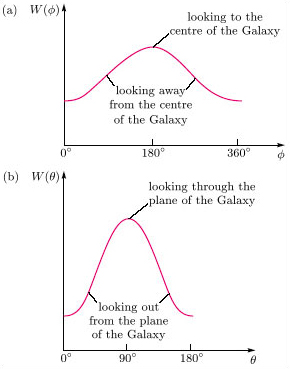Figure 18 Variation of intensity of radio waves with angle (a) in the plane of the Galaxy, and (b) in a plane perpendicular to the Galaxy

(a) If φ is the angle between the direction of the telescope and the line from the centre of the Galaxy to the telescope, with φ = 0 corresponding to looking directly away from the centre of the Galaxy, then, if the telescope is swept round in the plane of the Galaxy, the variation of intensity W of radio waves with φ will be that shown in Figure 18(a).

(b) If θ is the angle between the direction of the telescope and a line drawn perpendicular to the plane of the Galaxy at the telescope, with θ = 0 corresponding to looking directly ‘upwards’ through the disc, then the variation of intensity W of radio waves with θ will be as shown in Figure 18 (b).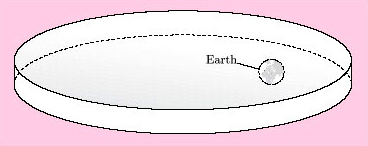Figure 19 The Earth inside the Galaxy, shown schematically as a disc

Having separated out these other sources of background noise, it was concluded that the isotropic component had to be of cosmic origin. It is called the cosmic microwave background radiation.

Figure 20(a) shows how complicated the overall microwave spectrum is, due largely to a set of lines generated in the Earth's atmosphere. Figure 20(b) focuses on the region at the left-hand side of Figure 20(a). This region is of interest because it is at lower frequencies than most of the atmospheric lines. The atmospheric interference varies with the thickness of the atmosphere, and hence with the angle of observation. This noise can therefore be separated out from the cosmic signal in which we are interested. Figure 20(b) shows the microwave spectrum after correcting for the effects of the atmosphere.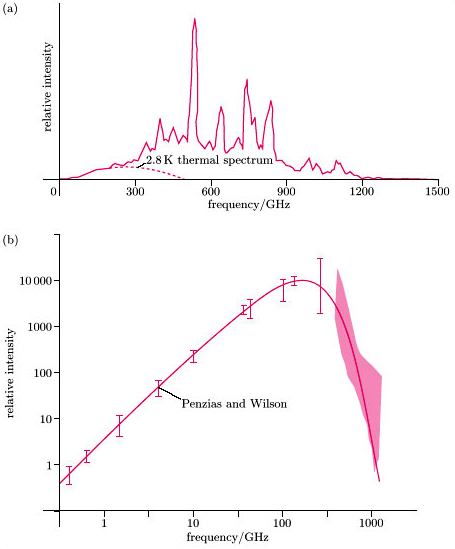(a) based on D.P. Woody et al. (1975) in Phys. Rev. Lett., 34, 1036–9; (b) based on R.A. Muller (1978) in Scientific American, 238, 64–74 ©
(a) based on D.P. Woody et al. (1975) in Phys. Rev. Lett., 34, 1036–9; (b) based on R.A. Muller (1978) in Scientific American, 238, 64–74
Figure 20 (a) The overall ground-based microwave spectrum. (b) Ground-based measurements by Penzias and Wilson, and by other observers, of the microwave intensity at various frequencies; an enlargement of the spectrum shown at the left-hand side of (a), corrected to remove the effects of the atmosphere. The shaded area represents the limits given by measurements made with a detector that covered a wide band of frequencies. (The solid line shows a thermal spectrum corresponding to a temperature of 2.8 K. The term ‘thermal spectrum’ is explained a little later in the text.)

In 1989, the COBE satellite was launched. It was able to make measurements from above the Earth's atmosphere and was therefore not subject to some of the problems encountered by Penzias and Wilson, and by other ground-based observers. However, a major contaminant – radiation originating from within the Galaxy – remained. The COBE results, after correction for this effect, are shown in Figure 21.

The shape revealed by this closer look is identical to that found for the spectrum inside a hot cavity – for example the spectrum in an oven or furnace (Figure 22). It is called a ‘black body’ spectrum (because it is that which is given out by an idealised heated black surface), or simply a ‘thermal’ spectrum.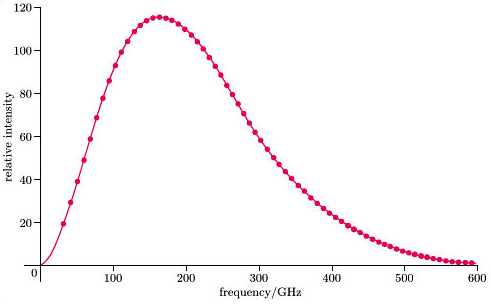courtesy of NASA/CSFC and COBE Science Working Group ©
courtesy of NASA/CSFC and COBE Science Working Group
Figure 21 Data on the microwave background radiation taken by the COBE satellite. The curve through the data points is that of a thermal spectrum of 2.73 K. Note that Figure 20(b) had a logarithmic frequency scale, while this one is linear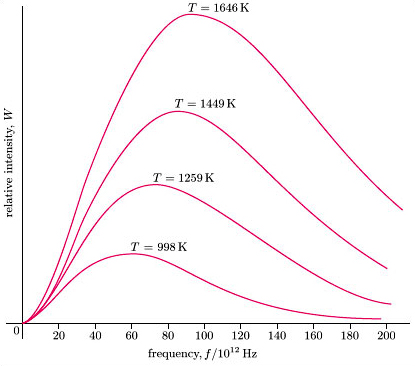Figure 22 Thermal spectra for various temperatures, T, based on laboratory measurements of relative intensity, W, of radiation with frequency f

One of the basic features of thermal radiation is that, regardless of the temperature of the surface or enclosure generating it, the shape of the spectrum is always the same. The peak of the spectrum moves up to higher frequencies as the temperature rises (it might glow red hot or white hot). But the basic shape remains the same. What this means is that if the spectra for two different temperatures are drawn on two graphs, we can always choose scales – linear but different – so that the graphs can be superimposed (Figures 23 and 24). This single, characteristic shape arises from, and is an indicator of, the equilibrium conditions inside the oven. The rescaling in Figure 23 works only for thermal spectra, and so is a true indicator of equilibrium.

As an example of the opposite extreme, take the line spectra from a hydrogen lamp and a sodium lamp. No amount of rescaling would fit these two together; they are quite distinct.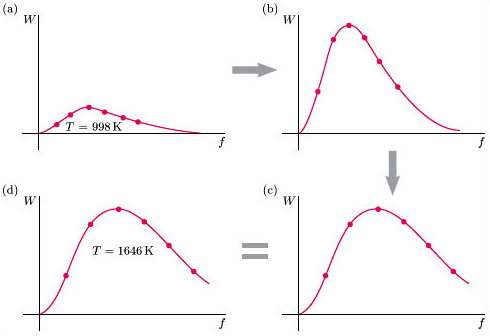Figure 23 What is meant by the shapes of thermal spectra being independent of temperature: (a) is the measured spectrum at 998 K (from Figure 22); (b) is obtained from (a) by expanding the vertical scale by a factor of (1646﻿/﻿998)﻿3; (c) is obtained from (b) by expanding the horizontal scale by a factor of (1646/998); (d) is the measured spectrum at 1646 K (from Figure 22) plotted using the same scales as those in (a), and is the same curve as (c).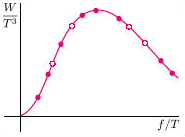Figure 24 As suggested by Figure 23, a plot of W/T3 as a function of f/T is a single curve, for all temperatures; that is, if W/T3 is plotted against f/T for a number of frequencies and temperatures, the plotted points for all frequencies and temperatures lie on one and the same curve

Since the cosmic microwave spectrum has a thermal shape; the conclusion is that it was generated in equilibrium conditions – in some sort of cavity. But the radiation fills the Universe: the ‘cavity’ is the Universe itself, a cavity with no walls.

The word ‘equilibrium’ is used here with some reservations. The Universe has not reached overall equilibrium, and strictly speaking never will. This is because of the expansion of the Universe. We saw in Section 4.1 how the wavelength of light from distant galaxies is redshifted due to the expansion of the Universe. It turns out that the same thing happens to the microwave background radiation; its wavelength is also increased. The peak frequency of the spectrum is reduced, and this means the radiation is progressively cooling. Hence the radiation has not strictly speaking reached equilibrium. However, the processes which transferred energy between radiation and matter in the early Universe were so rapid that it is meaningful to think of a quasi-equilibrium state having been attained at an early time, with the temperature gradually falling subsequently because of the expansion of the Universe.

A second feature of the thermal spectrum is that if the detector is situated within the ‘oven’ generating it (as distinct from looking at a distant black surface or opening to an oven), the intensity of the radiation at any particular frequency uniquely identifies the thermal curve to which it belongs, i.e. what the temperature of the ‘oven’ is. So, if Figure 22 referred to thermal spectra picked up by a detector situated inside an oven, the ordinate of the graph could be expressed in terms of absolute, rather than relative intensities. Under these circumstances, a measurement of intensity at a single frequency, say 60 × 1012 Hz, would be sufficient to identify which curve that data point belonged to, and hence what the temperature of the oven was.

Inasmuch as the Universe can be regarded as an ‘oven’, and we are in it, Penzias and Wilson were able to estimate from the intensity of the radiation at the single frequency they were detecting that the temperature was about 3 K. For this reason, the cosmic microwave background radiation is often called the 3 K radiation. The spectrum observed by COBE allowed a more precise estimate of the temperature, namely 2.73 K. This is based on the results presented in Figure 21, where it should be noted that the data points do not deviate from the thermal curve by more than 0.03%, this being consistent with measurement precision.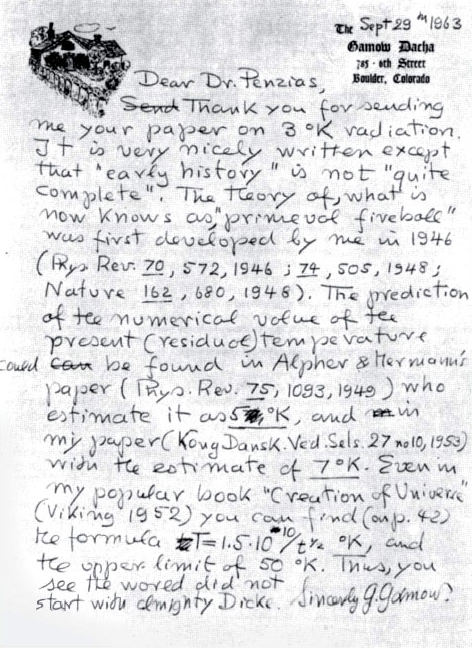courtesy of Dr A.A. Penzias ©
courtesy of Dr A.A. Penzias
Figure 25 A letter from George Gamow to Arno Penzias

The reference to ‘almighty Dicke’ at the end of this letter concerns R. H. Dicke, the leader of a group at Princeton University (also in New Jersey) which was pursuing both theoretical and observational research into the background radiation in the 1960s. The paper by Penzias and Wilson announcing their discovery, and a paper by Dicke's group providing a possible cosmological interpretation, were published back to back in volume 142 of the Astrophysical Journal in 1965.

The spectrum of thermal radiation at a given temperature T can be expressed in terms of a function W(f, T) which gives the intensity of radiation of frequency f. The energy density of that part of the radiation with frequencies lying between f and f + Δf is given by W(f, T) Δf. The formula for W(f, T) was derived by Max Planck in 1900: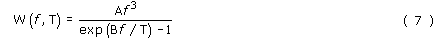where A and B are constants. At low frequencies (fT/B), one can use a simpler approximate formula: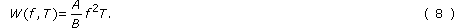### Study comment

We suggest that you do not omit Question 10 as it contains information that will be needed later.

### Question 10

(a) Verify the result represented by Figure 24, that W/T3 is a function of f/T only, which applies for all frequencies and temperatures.

(b) Use the result of (a) to show that maximum intensity occurs at a frequency, fmax, which is proportional to the temperature.

(c) Imagine that you receive a radio message from a very distant galaxy, informing you about measurements of the cosmic background radiation at various frequencies (defined in terms of fractions of the frequency of a standard spectral line). These results do not agree with your own measurements of the radiation. In particular, you find that the quoted value of the maximum intensity is eight times your value and occurs at a frequency that is twice your frequency for maximum intensity. You find that the quoted intensities at low frequencies are twice your values at the same frequencies. How can you explain these discrepancies? (You may assume that the discrepancy is not due to calibration or other errors.)

(a) Let y = W/T3 and x = f/T. Then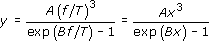which shows that W/T3 is a function of f/T only.

(b) From the above expression for y, we see there must be a universal value of x which gives a maximum value of y. But a maximum value of y implies a maximum value of W at a given temperature. If we denote this value of x by the constant xmax, it follows that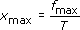and hence that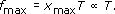Thus the frequency, fmax which gives maximum intensity is proportional to the temperature T. (You might have heard of this relation referred to as Wien's displacement law.)

(c) The explanation is that the cosmic background radiation has cooled during the time it took for the radio message to reach you. The discrepancies between their results and yours can all be explained by this difference in temperature of the radiation.

In part (b), it was shown that the frequency, fmax, corresponding to maximum intensity, is proportional to T. Since their value of fmax is twice yours, the temperature of the cosmic background radiation then must have been twice what it is here and now, i.e. it must have been just under 6 K.

In part (a), it was shown that: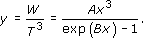If the maximum intensity is Wmax, it follows that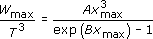which is independent of T since xmax is a universal constant from part (b). Hence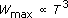which is why their value of Wmax is 23 = 8 times yours.

Finally, Equation 8 shows that the intensity at a given low frequency is proportional to the temperature and hence will be twice what you measure now at the same low frequency.

### Question 11

(a) On the basis of your solutions to parts (a) and (b) of Question 10, which would be hotter, a red star or a yellow star?

(b) The curves of Figure 22 can be used to extrapolate results to lower temperatures. By taking a measurement off the figure, and using the result of Question 10(b), estimate the frequency of the intensity maximum for radiation emitted by a body at room temperature. What is the term used to describe electromagnetic radiation in this frequency range?

(a) We have already established (in part (b) of the previous question) that the frequency of maximum intensity increases proportionately with temperature. As yellow light has a higher frequency than red light we would expect the yellow star to be the hotter.

(b) From Figure 22, we see that the maximum intensity for a temperature of, say, 1646 K occurs at a frequency of about 92 × 1012 Hz. The maximum for room temperature, taken to be 300 K, is then given by (300/1646) × 92 × 1012 Hz = 16.8 × 1012 Hz.

Radiation of this frequency occurs in the infrared part of the spectrum.

S357_1